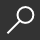## Introduction to Probability, Statistics, and Random Processes by Hossein Pishro-Nik

Overview -

This book introduces students to probability, statistics, and stochastic processes. It can be used by both students and practitioners in engineering, various sciences, finance, and other related fields. It provides a clear and intuitive approach to these topics while maintaining mathematical accuracy.

The book covers:

• Basic concepts such as random experiments, probability axioms, conditional probability, and counting methods
• Single and multiple random variables (discrete, continuous, and mixed), as well as moment-generating functions, characteristic functions, random vectors, and inequalities
• Limit theorems and convergence
• Introduction to Bayesian and classical statistics
• Random processes including processing of random signals, Poisson processes, discrete-time and continuous-time Markov chains, and Brownian motion
• Simulation using MATLAB and R (online chapters)

The book contains a large number of solved exercises. The dependency between different sections of this book has been kept to a minimum in order to provide maximum flexibility to instructors and to make the book easy to read for students. Examples of applications-such as engineering, finance, everyday life, etc.-are included to aid in motivating the subject. The digital version of the book, as well as additional materials such as videos, is available at www.probabilitycourse.com.

local_shippingFor DeliveryIn Stock.
This item is Non-Returnable.
FREE Shipping for Club Members helpNew & Used Marketplace 12 copies from \$25.45

## More About Introduction to Probability, Statistics, and Random Processes by Hossein Pishro-Nik

### Overview

This book introduces students to probability, statistics, and stochastic processes. It can be used by both students and practitioners in engineering, various sciences, finance, and other related fields. It provides a clear and intuitive approach to these topics while maintaining mathematical accuracy.

The book covers:

• Basic concepts such as random experiments, probability axioms, conditional probability, and counting methods
• Single and multiple random variables (discrete, continuous, and mixed), as well as moment-generating functions, characteristic functions, random vectors, and inequalities
• Limit theorems and convergence
• Introduction to Bayesian and classical statistics
• Random processes including processing of random signals, Poisson processes, discrete-time and continuous-time Markov chains, and Brownian motion
• Simulation using MATLAB and R (online chapters)

The book contains a large number of solved exercises. The dependency between different sections of this book has been kept to a minimum in order to provide maximum flexibility to instructors and to make the book easy to read for students. Examples of applications-such as engineering, finance, everyday life, etc.-are included to aid in motivating the subject. The digital version of the book, as well as additional materials such as videos, is available at www.probabilitycourse.com.

This item is Non-Returnable.

### Details

• ISBN-13: 9780990637202
• ISBN-10: 0990637204
• Publisher: Kappa Research, LLC
• Publish Date: August 2014
• Page Count: 746
• Dimensions: 9.69 x 7.44 x 1.49 inches
• Shipping Weight: 2.88 poundsRelated Categories